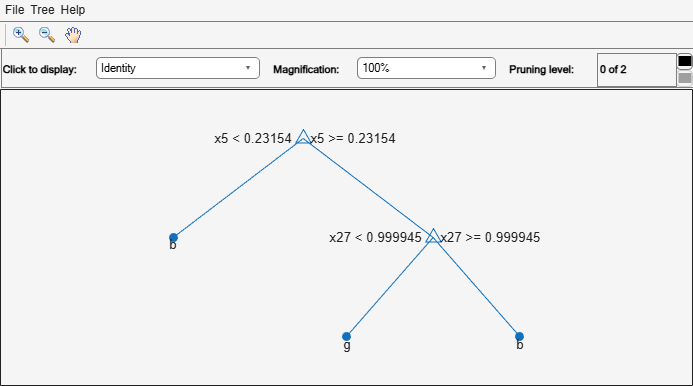# cvloss

Class: ClassificationTree

Classification error by cross validation

## Syntax

``E = cvloss(tree)``
``````[E,SE] = cvloss(tree)``````
``````[E,SE,Nleaf] = cvloss(tree)``````
``````[E,SE,Nleaf,BestLevel] = cvloss(tree)``````
``[___] = cvloss(tree,Name,Value)``

## Description

````E = cvloss(tree)` returns the cross-validated classification error (loss) for `tree`, a classification tree. The `cvloss` method uses stratified partitioning to create cross-validated sets. That is, for each fold, each partition of the data has roughly the same class proportions as in the data used to train `tree`.```
``````[E,SE] = cvloss(tree)``` returns the standard error of `E`.```
``````[E,SE,Nleaf] = cvloss(tree)``` returns the number of leaves of `tree`.```
``````[E,SE,Nleaf,BestLevel] = cvloss(tree)``` returns the optimal pruning level for `tree`.```
````[___] = cvloss(tree,Name,Value)` cross validates with additional options specified by one or more `Name,Value` pair arguments, using any of the previous syntaxes. You can specify several name-value pair arguments in any order as `Name1,Value1,…,NameN,ValueN`.```

## Input Arguments

expand all

Trained classification tree, specified as a `ClassificationTree` model object produced by `fitctree`.

### Name-Value Arguments

Specify optional comma-separated pairs of `Name,Value` arguments. `Name` is the argument name and `Value` is the corresponding value. `Name` must appear inside quotes. You can specify several name and value pair arguments in any order as `Name1,Value1,...,NameN,ValueN`.

Pruning level, specified as the comma-separated pair consisting of `'Subtrees'` and a vector of nonnegative integers in ascending order or `'all'`.

If you specify a vector, then all elements must be at least `0` and at most `max(tree.PruneList)`. `0` indicates the full, unpruned tree and `max(tree.PruneList)` indicates the completely pruned tree (i.e., just the root node).

If you specify `'all'`, then `cvloss` operates on all subtrees (i.e., the entire pruning sequence). This specification is equivalent to using `0:max(tree.PruneList)`.

`cvloss` prunes `tree` to each level indicated in `Subtrees`, and then estimates the corresponding output arguments. The size of `Subtrees` determines the size of some output arguments.

To invoke `Subtrees`, the properties `PruneList` and `PruneAlpha` of `tree` must be nonempty. In other words, grow `tree` by setting `'Prune','on'`, or by pruning `tree` using `prune`.

Example: `'Subtrees','all'`

Data Types: `single` | `double` | `char` | `string`

Tree size, specified as one of the following values:

• `'se'``cvloss` uses the smallest tree whose cost is within one standard error of the minimum cost.

• `'min'``cvloss` uses the minimal cost tree.

Example: `'TreeSize','min'`

Number of cross-validation samples, specified as a positive integer value greater than 1.

Example: `'KFold',8`

## Output Arguments

expand all

Cross-validation classification error (loss), returned as a vector or scalar depending on the setting of the `Subtrees` name-value pair.

Standard error of `E`, returned as a vector or scalar depending on the setting of the `Subtrees` name-value pair.

Number of leaf nodes in `tree`, returned as a vector or scalar depending on the setting of the `Subtrees` name-value pair. Leaf nodes are terminal nodes, which give classifications, not splits.

Best pruning level, returned as a scalar value. By default, a scalar representing the largest pruning level that achieves a value of `E` within `SE` of the minimum error. If you set `TreeSize` to `'min'`, `BestLevel` is the smallest value in `Subtrees`.

## Examples

expand all

Compute the cross-validation error for a default classification tree.

Load the `ionosphere` data set.

`load ionosphere`

Grow a classification tree using the entire data set.

`Mdl = fitctree(X,Y);`

Compute the cross-validation error.

```rng(1); % For reproducibility E = cvloss(Mdl)```
```E = 0.1168 ```

`E` is the 10-fold misclassification error.

Apply k-fold cross validation to find the best level to prune a classification tree for all of its subtrees.

Load the `ionosphere` data set.

`load ionosphere`

Grow a classification tree using the entire data set. View the resulting tree.

```Mdl = fitctree(X,Y); view(Mdl,'Mode','graph')```Compute the 5-fold cross-validation error for each subtree except for the highest pruning level. Specify to return the best pruning level over all subtrees.

```rng(1); % For reproducibility m = max(Mdl.PruneList) - 1```
```m = 7 ```
`[E,~,~,bestLevel] = cvloss(Mdl,'SubTrees',0:m,'KFold',5)`
```E = 8×1 0.1282 0.1254 0.1225 0.1282 0.1282 0.1197 0.0997 0.1738 ```
```bestLevel = 6 ```

Of the `7` pruning levels, the best pruning level is `6`.

Prune the tree to the best level. View the resulting tree.

```MdlPrune = prune(Mdl,'Level',bestLevel); view(MdlPrune,'Mode','graph')```## Alternatives

You can construct a cross-validated tree model with `crossval`, and call `kfoldLoss` instead of `cvloss`. If you are going to examine the cross-validated tree more than once, then the alternative can save time.

However, unlike `cvloss`, `kfoldLoss` does not return `SE`,`Nleaf`, or `BestLevel`. `kfoldLoss` also does not allow you to examine any error other than the classification error.